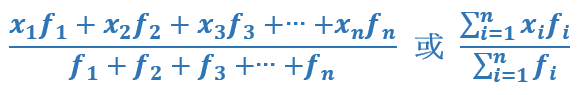Each observation is multiplied with a corresponding weight. The weighted mean is the sum of products divided by the sum of weights. In the formula, xi is the value of variable x and fi is the weight for x. In the formula, xi is the value of variable x and fi is the weight for x.

#### To calculate the weighted mean, please enter the corresponding weights of the values separated by comma ",".

Number of values: 0 Weighted mean: 0